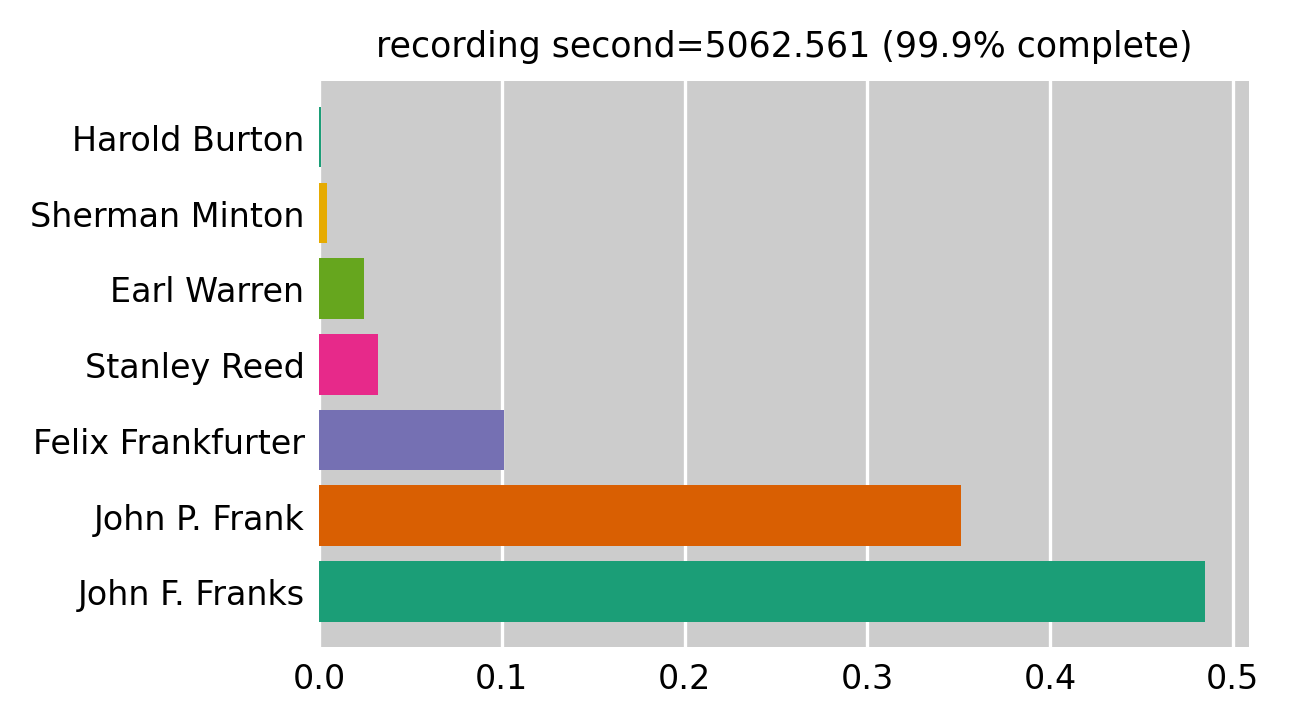## Setting the data directories and choosing an example transcript to work with

```import os
data_root = '/ws/data/voice-identity/supreme-court'
transcript_dirpath = os.path.join(data_root, 'transcripts')
audio_dirpath = os.path.join(data_root, 'full_audios')
ls = [f for f in sorted(os.listdir(transcript_dirpath)) if f != '.']
os.path.join(transcript_dirpath, ls)
```
`'/ws/data/voice-identity/supreme-court/transcripts/12989_transcript.pickle'`

## Gets the necessary data into variables

```from prcvd.models.oyez import OyezAudioDataBunch

db = OyezAudioDataBunch()
db.create(
transcript_dirpath=transcript_dirpath,
audio_dirpath=audio_dirpath,
url='https://apps.oyez.org/player/#/roberts10/oral_argument_audio/13101'
)
```

```db.bunch_sections.transcript_section.section
```
```{'raw': <p class="ng-binding ng-scope ng-isolate-scope" ng-class="{'active': sync.synced, 'search-result': player.isSearchResult(textBlock), 'clipping-active': player.drawer == 'clip', 'clipped': player.isClipped(textBlock)}" ng-click="player.onTextClick(textBlock)" ng-repeat="textBlock in turn.text_blocks" oyez-scroll-to="sync.synced || player.isCurrentSearchResult(textBlock)" oyez-sync="" start-time="0" stop-time="13.064" sync-queue="textBlocks"> Number 523, Joan Greenway Collins et al., versus American Buslines Incorporated, Respondent Employer. </p>,
'case_name': 'Collins v. American Buslines, Inc.',
'conv_date': datetime.datetime(1956, 3, 29, 0, 0),
'speaker': 'Earl Warren',
'start_time': 0.0,
'stop_time': 13.064,
'transcript': ' Number 523, Joan Greenway Collins et al., versus American Buslines Incorporated, Respondent Employer. '}```

```import matplotlib.pyplot as plt
import pandas as pd

from prcvd.talk_time_pipeline import (compute_talk_time, compute_prop_talk_time, compute_rolling_talk_timer,
nice_axes, compute_speaker_stats)

speaker_stats = compute_speaker_stats(databunch=db)
rtt,rpt = compute_rolling_talk_timer(
sorted_transcript=db.transcript.transcript,
speakers_seed=list(speaker_stats.keys())
)

df = pd.DataFrame(rpt)
df.index = df['ts']
del df['ts']

ts_perc = 0.999
ts = df.index.values[round(len(df)*ts_perc)]
fig, ax = plt.subplots(figsize=(4, 2.5), dpi=300)
colors = plt.cm.Dark2(range(6))
s = df.loc[ts]
y = s.index
width = s.values
ax.barh(y=y, width=width, color=colors);
ax.set_title('recording second={} ({}% complete)'.format(ts, ts_perc*100), fontsize='smaller')

nice_axes(ax)
``````from random import randrange
from ncls import NCLS

class UnlabeledSection:
def __init__(self, start_sec, close_sec, audio_data):
self.start_sec = start_sec
self.close_sec = close_sec
self.audio_data = audio_data

self.start_tsf = self._tsf_from_sec(
sec=self.start_sec, sample_rate=self.audio_data.sample_rate
)
self.close_tsf = self._tsf_from_sec(
sec=self.close_sec, sample_rate=self.audio_data.sample_rate
)

def _tsf_from_sec(self, sec, sample_rate):
return sec * sample_rate

def get_start_stop(start_sec, close_sec, sample_rate):
"""
Discretizes the float (second time) index to sample index

"""
ss = int(start_sec * sample_rate) + 1
cs = int(close_sec * sample_rate)
return ss, cs

def sample_from_waveform(audio_data, sample_len):
close_sec = randrange(
round(round(audio_data.waveform.shape / audio_data.sample_rate) - sample_len)
)
start_sec = close_sec - sample_len
return UnlabeledSection(
start_sec=start_sec,
close_sec=close_sec,
audio_data=audio_data.slice_waveform(start_sec=start_sec, close_sec=close_sec)
)

```

```def get_ncls_format(ordered_transcript, sample_rate):
time_indexed_sections = {}
starts = []
ends = []
index = []
for section in ordered_transcript:
ss, cs = get_start_stop(
start_sec=section.section['start_time'],
close_sec=section.section['stop_time'],
sample_rate=sample_rate
)
starts.append(ss)
ends.append(cs)
index.append(cs)
time_indexed_sections[cs] = section

return time_indexed_sections, starts, ends, index
```

```time_indexed_sections, starts, ends, index = get_ncls_format(
ordered_transcript=db.transcript.transcript, sample_rate=db.audio_data.sample_rate
)
```

```import numpy as np
ints = NCLS(starts=np.array(starts), ends=np.array(ends), ids=np.array(index))
s1 = sample_from_waveform(audio_data=db.audio_data, sample_len=10.0)
```

## Planning for model architecture

"We show that artificially augmenting the training data with noises and reverberation is a highly effective strategy for improving performance in DNN embedding systems." Action: create functions that allow for waveform noise and reverb (what kind of noise? what kind of reverb?)

## Example that verifies that the audio is loaded correctly.

```import IPython

audios = [fp for fp in os.listdir(audio_dirpath) if fp != '.']
IPython.display.Audio(os.path.join(audio_dirpath, audios),
rate=sample_rate)
```